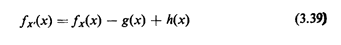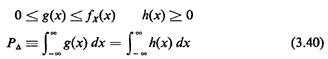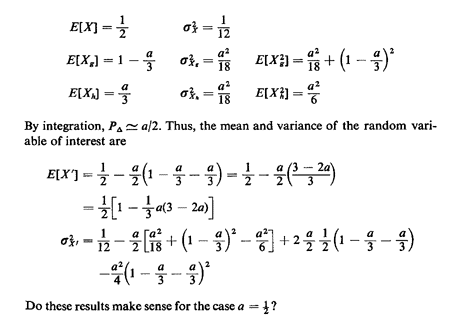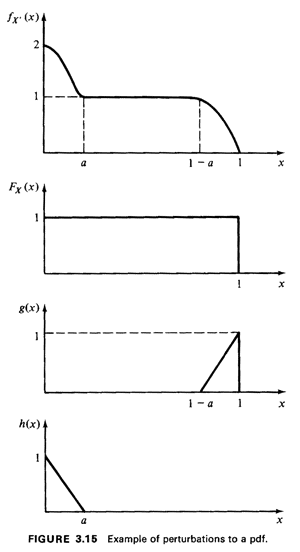## 3.2.2 Perturbations to a PDF

Sometimes use of perturbation ideas may help us to approximate a complicated pdf in terms of simpler quantities. Suppose thatfx'(x) is the pdf of interest. WritewhereHere fx(x) is the "simpler" pdf ; h(x) is an added and g(x) a subtracted perturbation term. The perturbation functions, when normalized by P, may themselves be considered to be conditional pdf's. Xg is the random variable having pdf g(x)/Pand Xh is the random variable having pdf h(x)/P. Then, the expected value for the random variable of interest isExample 7: Triangle Pertarbations Consider the example illustrated in Figure 3.15. The "complicated" pdf fx'(x) may be approximated as the sum of a uniform pdf and a triangular function h(x), with the triangular function g(x) subtracted from the sum. From previous work we know the following: